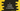# C program to arrange numbers in ascending order## C program to arrange numbers in ascending order :

This C programming tutorial will show you how to arrange user provided numbers in ascending order. We will write one program that will take random amount of numbers from the user and print them in ascending order.

We will write different functions to print the array and to sort the array numbers in ascending order.

With this post, you will learn how to take user inputs and store them in array, how to iterate through an array and how to modify an array.

### C Program:

Below is the complete c program that arranges user given numbers in ascending order.

``````#include <stdio.h>

void printArray(int *arr, int size)
{
int i;

for (i = 0; i < size; i++)
{
printf("%d ", arr[i]);
}
printf("\n");
}

void sortInAscendingOrder(int *arr, int size)
{
int i, j, temp;

for (i = 0; i < size; i++)
{
for (j = i + 1; j < size; j++)
{
if (arr[i] > arr[j])
{
temp = arr[i];
arr[i] = arr[j];
arr[j] = temp;
}
}
}
}

int main()
{
int arraySize, i;

printf("Enter total count of numbers :");
scanf("%d", &arraySize);

int arr[arraySize];

for (i = 0; i < arraySize; i++)
{
printf("Enter value :");
scanf("%d", arr + i);
}

printf("Before sort :");
printArray(arr, arraySize);

sortInAscendingOrder(arr, arraySize);

printf("After sort :");
printArray(arr, arraySize);
}``````

### Explanation:

Let me explain the above program :

1. We are asking the user to enter the total count for the array. We are storing that value in the variable arraySize and creating one integer array of size arraySize.
2. Using one for loop, we are reading the user input values.
3. We are calling printArray function to print the content of the array. This function iterates through the given array and prints them one by one.
4. sortInAscendingOrder function sorts the array contents in ascending order. We are using two for loops in this function. The outer for loop iterates from left to right one by one. For each element that is pointed by the outer loop, the inner loop compares it with all elements to the right of it. The inner loop finds the smallest element and replaces it with the element pointed by the outer loop.

i.e. on each iteration of the outer loop, we are picking the smallest element to the right of the outer loop and placing it at front.

### Sample Output:

``````Enter total count of numbers :5
Enter value :15
Enter value :2
Enter value :24
Enter value :100
Enter value :1
Before sort :15 2 24 100 1
After sort :1 2 15 24 100

Enter total count of numbers :5
Enter value :1
Enter value :2
Enter value :3
Enter value :4
Enter value :5
Before sort :1 2 3 4 5
After sort :1 2 3 4 5 ``````